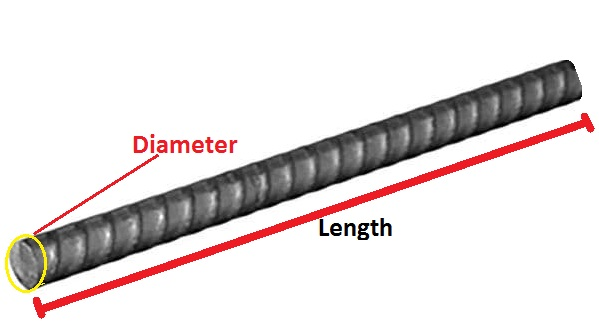# How to calculate weight of steel bar dia 8mm, 10mm, 12mm, 16mm- Excell

## Input detail of Rebar### Rebar (A)

.......................................................

### Rebar (B)

.......................................................

## How civil rod weight calculator works?

Hello, guys here I am going to give you the most important answer to the question which is most useful in the field of civil engineering. I will show you here how to calculate weight of steel bar dia 8mm and its process with formula.

The standard size of steel bars used in the construction of different structures like a building, bridge, culvert, etc. are 6mm, 8mm, 10mm, 12mm, 16mm, 20mm, 25mm, 28mm, 32mm, 36mm, and 40mm.

## Standard size of steel bars and weight of steel bar per meter

Besides this, contractors have to order the manufacturing company to make the steel rod having a specific diameter if required.

So, let’s start.

First of all we have to know that,

Density of any materials is given by,

Density = (Mass/Volume)

So, Mass = Density x Volume

From here we can conclude that, if we can know about the density and volume of steel then we can obviously calculate the mass or weight of the steel rod.

Let us calculate one by one.

We know that the density of steel is 7850 kg/meter cube

And Since, the steel are in cylindrical shape we can conclude a steel rod as a cylinder.

So, the volume of cylinder is given by,

Where, V = Volume of required steel rod.

L = Length of required steel rod.

D = Diameter of steel rod.

Now,

We have,

Mass = Density x Volume

(Leaving the unit of length in meter and diameter in millimeter.)

We will get,

Now, just put the value of length of steel rod in meter and diameter in millimeter you will get the weight of steel rod.

So, Our single formula to calculate weight of steel rod becomes.

If I take length of steel rod one meter.

Then,

It means that equation (1) becomes the weight of steel rod per meter.

Just we have to put the value of diameter of steel rod.

## Q) Calculate weight of 10 mm tmt bar(10 mm saria weight)having length 8 meter.

So, we have,

or, Weight = (10*10/162.25)*8

(Remember that I have put the value of length in meter and diameter in millimeter.)

Now, by calculating above expression we will get,

Mass = 4.93 kg (Approx.)

## Q) Calculate 12 mm tmt bar weighthaving length 8 meter.

So, we have,

(Remember that I have put the value of length in meter and diameter in millimeter.)

Now, by calculating above expression we will get,

Mass = 7.1 kg (Approx.)

## Q) Calculate 16mm tmt bar weight having a length of 8 meters.

So, we have,

or, Weight = (16*16/162.25)*8

(Remember that I have put the value of length in meter and diameter in millimeter.)

Now, by calculating above expression we will get,

Mass = 12.62 kg (Approx.)

## Q) Calculate 20mm rod weighthaving length 8 meter.

So, we have,

= (20*20/162.25)*8

(Remember that I have put the value of length in meter and diameter in millimeter.)

Now, by calculating above expression we will get,

Mass = 19.72 kg (Approx.)

Hence, In this way, we can calculate the weight of steel rod per meter of different diameters having different lengths.

## Weight of steel rod per feet

In the same way, we can calculate the weight of steel rod per feet having different diameters in millimeters.

The Single formula to calculate the unit weight of steel rod per feet is given by,

But, Length is in feet.

So, according to unit conversion we will get,

## Q) Calculate 8mm steel rod weight 40 ft(8mm saria weight)

So, we have,

= (8*8/533)*40

(Remember that I have put the value of length in feet and diameter in millimeter.)

Now, by calculating above expression we will get,

Mass = 4.80 kg (Approx.)

## Q) Calculate 40 feet 12mm rod weight

So, we have,

= (12*12/533)*40

(Remember that I have put the value of length in feet and diameter in millimeter.)

Now, by calculating above expression we will get,

Mass = 10.80 kg (Approx.)

## Q) Calculate the weight of 32mm dia steel bar of 40 feet.

So, we have,

= (32*32/533)*40

(Remember that I have put the value of length in feet and diameter in millimeter.)

Now, by calculating above expression we will get,

Mass = 76.84 kg (Approx.)

## Watch video for How to calculate weight of steel bar dia 8mm , 10mm, 12mm, and so on..,

I hope this article "How to calculate weight of steel bar dia 8mm, 10mm, 12mm, 16mm" remains helpful for you.

Happy Learning - Civil Concept

Contributed by,

Civil Engineer - Ranjeet Sahani